## How to Interface CH926 Multi Coin Acceptor with Arduino UNO

Keeping track of coins and counting their balance can be a hassle. You have to remember how many coins you have of each denomination, and then you have to count them all up to get your total balance. I will never forget the day I realized just how much time I was wasting counting coins. I was exhausted after yet another counting session, and I knew there had to be a simpler way. I decided to build a multi-coin acceptor that would count my coins for me. This project was a great learning experience, and it also saved me a lot of time.

A multi-coin acceptor is a device that can accept up to 6 different types of coins. It is connected to an LCD, which shows the total balance of all the coins that have been inserted. This makes it easy to keep track of your coins and to know how much money you have available.

Let us begin this exciting project! We will be learning about coin recognition, microcontroller programming, and interfacing of the LCD.

## Circuit DiagramConnections:

• Connect the positive of the Coin Acceptor to the positive terminal of the 12V battery, and its GND pin to the breadboard.
• Connect the negative terminal of the battery to the breadboard such that the negative terminal of the battery and GND of the coin acceptor are serially connected.
• Connect the GND of Arduino UNO and I2C in the same manner such that all the components have the same ground to avoid excessive wired connections.
• Connect the Coin pin of the Coin Acceptor to the 5V pin of Arduino Uno using a 10k pullup resistor. On the other end, connect Digital Pin 2 of the Arduino UNO.
• Connect all the pins of LCD to the I2C module and interconnect the backlight jumper pins as shown
• Connect the VCC pin of the I2C module to the 5V pin, the SCL pin to the A5 pin and the SDA pin to the A4 pin of the Arduino UNO.

That’s it for the connections, once this is done you can see that the Coin Acceptor will be ON.

The connections should look like this: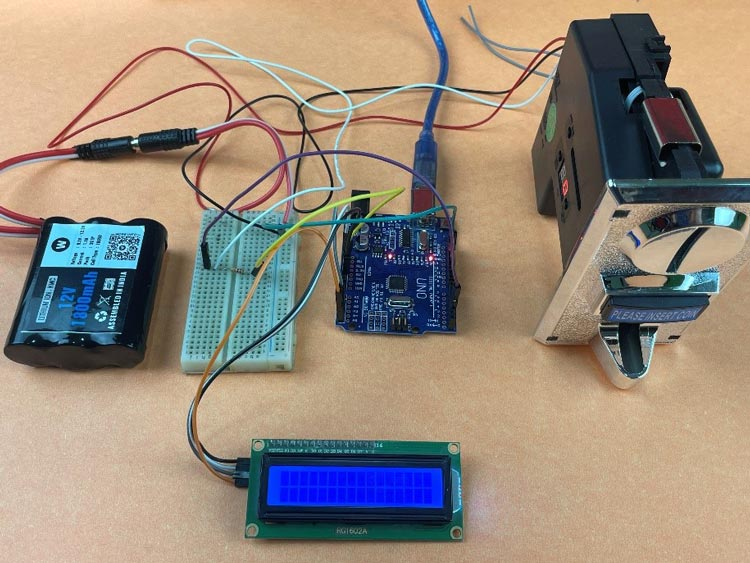Now, let us see how the Coin Acceptor Works and how can we train it so that it correctly detects the value of inserted coins.

## Multi Coin Acceptor Module CH-926Specifications:

• Identify accuracy rate: 95%
• Diameter: Ф15mm-Ф29mm
• Thickness: 1.8mm-3.0mm
• Operating voltage: DC12V ± 10%
• Working current: 50mA
• Recognition speed: ≤0.6sec
• Dimensions: 6.3 x 6 x 12.3 cm

The CH-926 Coin acceptor is a device that is widely used in different applications, including vending machines, arcade games, and self-service devices, due to its versatility and reliability. It uses both weight and size to accurately identify coins. When a coin is inserted into the acceptor, it lands on a piezoelectric element, which produces an electrical pulse corresponding to its weight. At the same time, the acceptor also employs a sensor to measure the diameter of the coin.
The acceptor can be programmed to accept up to 6 different types of coins and utilizes the weight and size data to ascertain the denomination of the coin. For instance, a 2-rupee coin will produce a stronger electrical pulse and possess a greater diameter compared to a 1-rupee coin. Subsequently, the acceptor transmits this data to a microcontroller, which determines the worth of the coin.

Not only does the microcontroller perform coin denomination calculations, but it also has control over the acceptor's output. Upon accepting a coin, the acceptor emits a pulse which can be utilized to activate different devices like solenoids.

## Training Coin Acceptor Module

• Press and hold the ADD and MINUS buttons together for 1 second. This will enter the training mode. The 0 on the 7-segment display will change to A.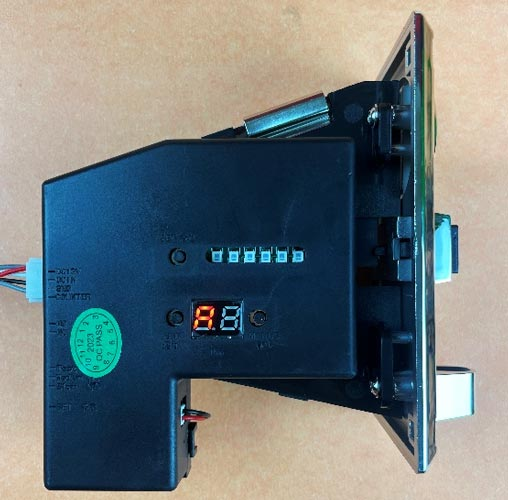• Press the SET button once. This will change the A on the 7-segment display to E. The E indicates that you are now setting the number of different coins you have. The number can range from 1 to 6. You can use the ADD and MINUS buttons to increase or decrease the number. If you set the number to 6, the module will be able to recognize 6 types of coins.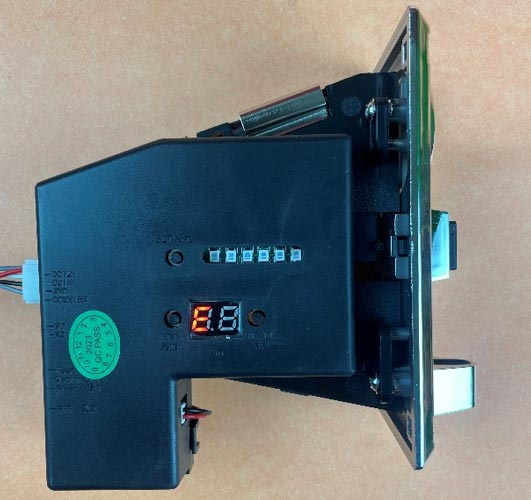• Once you have entered the desired number, press the SET button again. This will change the E on the 7-segment display to H1, and the first SMD LED will be glowing. H denotes the number of samples which can range from 1 to 20. Press the ADD button to increase the number of samples you want to send, or the MINUS button to decrease the number.  It is recommended to use at least 15 samples so that the coins are detected accurately.• Once you have selected the number of samples, such as 20, press SET again. Now, the 7 segment displays P1. P represents the value assigned to the coin. If you are using a 1 Re coin, set it to 1. If you are training for a 10 Rs coin, set P to 10, and so on.• After assigning the desired value on the 7-segment display for the coin, press the "SET" button once more. At this point, you will observe that the display switches to "F1". In this particular situation, the letter "F" symbolizes the level of precision or accuracy in detecting the coin by the module. The precision value, indicated by "F," can vary from 1 to 20. A smaller "F" value suggests a greater degree of accuracy. As per the user instruction document, it is suggested to set the precision value to 8. This value strikes a balance between accuracy and performance.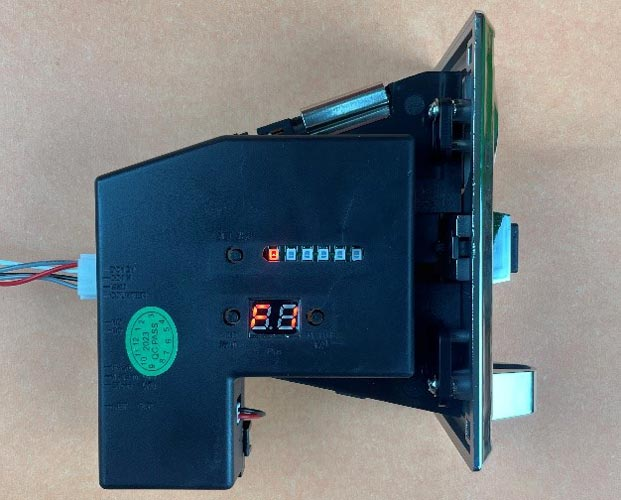• After pressing the "SET" button to set the precision value (F), press the button once more. The display will then indicate "H2" and the second SMD LED will be glowing. During this step, there is no requirement to alter the values of H and F; they can stay unchanged from before. Now, concentrate on assigning values to the coin parameter (P) according to your needs. You have the flexibility to change the values of P for different coins that you wish to train the module to identify. Nevertheless, it is important to maintain the consistency of values for H and F throughout this procedure to ensure accuracy in detecting the coins.
• Repeat the same steps till you have trained the module for all the values. Say you set the value of E to 6, repeat the process till you set the value for F6 and press SET.
• After you have finished the previous steps, you will observe that all the SMD LEDs flash at the same time, and the display indicates "0". Proceed to press the "SET" button at this moment. The display will then switch to "A". Press the "SET" button once more, and the display will exhibit "A1" while the initial SMD LED lights up.• You are now prepared to send the samples for your initial coin. Keep in mind that the parameters for this coin are "H1" (previously established), "P1" (assigned value), and "F1" (precision value). Begin gathering and submitting the samples that depict this specific coin to the module for training.
• If you have assigned a value of 20 to H1 and 1 to P1, it indicates that you are training the module to identify a 1 Rupee coin. To collect samples for this coin, you must insert a 1 Rupee coin into the coin acceptor module 20 times. Once you have finished collecting the necessary samples for the initial coin, the display will exhibit "A2". This signifies that you should now submit samples for the second coin.
• Follow the same procedure for the remaining coins that you wish to train the module to identify. Keep repeating this process until you have collected samples for all the desired coins. The display will then increase to A3, A4, and so forth, indicating the advancement to the next coin in the training phase.

To verify if all the coins you tested are being recognized, follow these steps:

1. Insert the coins into the machine.
2. Look at the number displayed on the 7-segment display.
• If the displayed number is correct, it means that the machine has been trained correctly.
• If the displayed number is incorrect or the machine returns the coin through the "Rejection Coin Outlet," it means that the coin was not sampled properly.

Please be aware that any coins that were not sampled correctly or any coins that differ from the ones initially sampled will be returned through the rejection outlet.

Now that we have trained the module, let’s connect it to the Arduino UNO and LCD so that we can have the total amount deposited displayed on the LCD. You can refer to the connections explained in the above section to understand how to connect the Arduino to the Coin Acceptor and LCD.

## Displaying Total Balance on LCD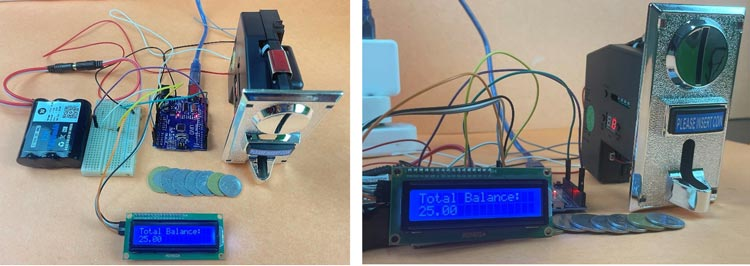Arduino Code For Multi Coin Acceptor

Understanding the Code:

const int coinPin = 2;

volatile int impulsCount = 0;

int coinType = 0;

float totalAmount = 0.0;

float coinValue;

This code defines the variables that will be used in the program. The coinPin variable is the pin that is connected to the coin acceptor. The impulsCount variable is a volatile variable that is used to count the number of pulses from the coin acceptor. The coinType variable is used to keep track of the type of coin that has been inserted. The totalAmount variable is used to keep track of the total amount of money that has been inserted. The coinValue variable is used to store the value of the coin that has been inserted.

const int coinPulses[] = {1, 2, 5, 10, 10};

const float coinValues[] = {1.0, 2.0, 5.0, 10.0, 10.0};

This code defines the arrays that are used to store the number of pulses and the value of each coin. The coinPulses array stores the number of pulses for each coin. The coinValues array stores the value of each coin.

int lookup(int pulses) {

for (int i = 0; i < sizeof(coinPulses) / sizeof(coinPulses); i++) {

if (pulses == coinPulses[i]) {

return coinValues[i];

}

}

return -1;

}

This code defines the lookup() function, which is used to look up the value of a coin based on the number of pulses. The function takes the number of pulses as input and returns the value of the coin.

void setup() {

Serial.begin(9600);

attachInterrupt(digitalPinToInterrupt(coinPin), coinInterrupt, FALLING);

totalAmount = 0.0;

EEPROM.write(0, 0);

lcd.init();

lcd.clear();

lcd.backlight();

}

This code defines the setup() function, which is called once when the Arduino is started. The function initializes the serial port, the interrupt, the total amount, the LCD display, and the backlight.

void coinInterrupt() {

impulsCount++;

}

This code defines the coinInterrupt() function, which is called whenever the coin acceptor detects a coin. The function increments the impulsCount variable.

void loop() {

if (impulsCount == coinPulses[coinType]) {

coinValue = lookup(impulsCount);

if (coinValue > 0) {

totalAmount += coinValue;

}

delay(100);

Serial.print("Total Amount: ");

Serial.println(totalAmount, 2);

impulsCount = 0;

lcd.setCursor(0, 0);

lcd.print("Total Balance: ");

lcd.setCursor(0, 1);

lcd.print(totalAmount, 2);

}

}

This code defines the loop() function, which is called repeatedly until the Arduino is turned off. The function checks if the impulsCount variable is equal to the number of pulses for a particular coin. If it is, the function then calculates the value of the coin and adds it to the total amount. The function then prints the total amount to the serial port and the LCD display. The impulsCount variable is then reset to 0.

## Conclusion

In conclusion, the CH-926 Coin Acceptor provides a dependable and effective solution for coin management. Its capacity to accurately detect coins using weight and size, as well as its programmability for multiple coin types, makes it a versatile option for different uses. Additionally, its integration with a microcontroller and LCD display enhances its functionality by offering immediate feedback and effortless tracking of overall balances.